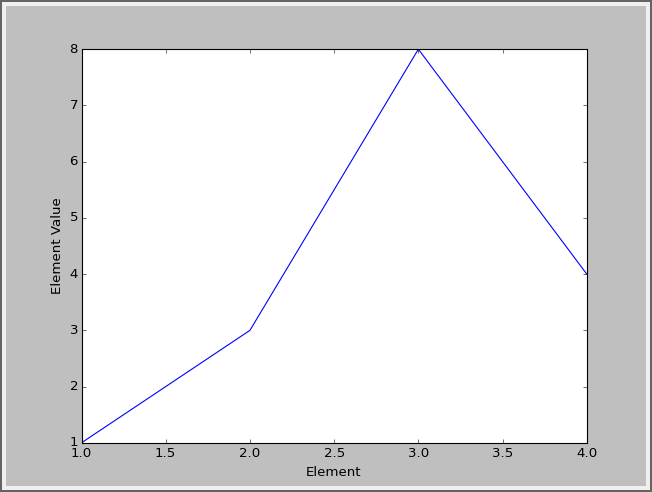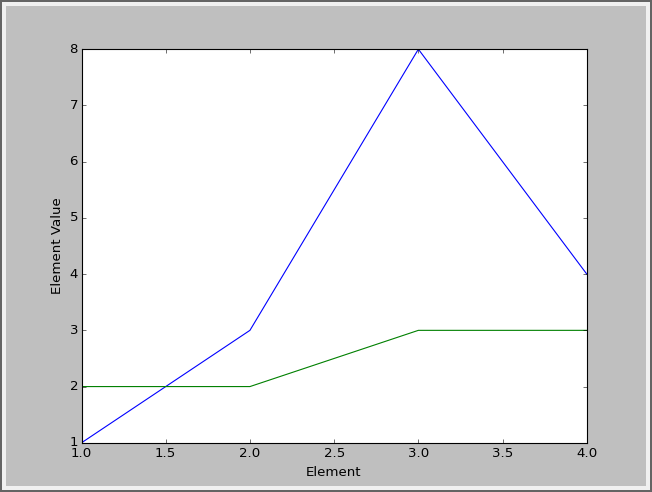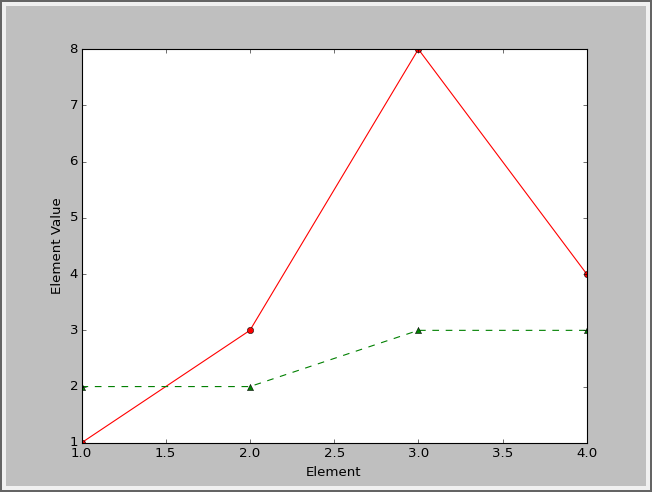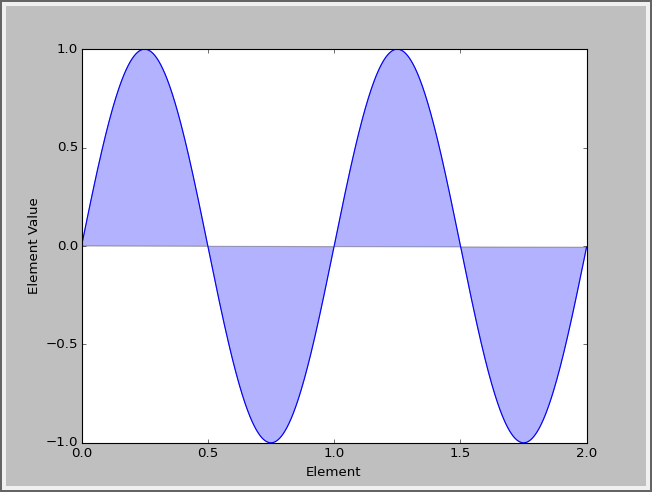﻿ Program Arcade Games With Python And Pygame

# Program Arcade GamesWith Python And Pygame

 < Previous Home Next >

# Chapter 14: Libraries and Modules

A library is a collection of code for functions and classes. Often, these libraries are written by someone else and brought into the project so that the programmer does not have to “reinvent the wheel.” In Python the term used to describe a library of code is module.

By using import pygame and import random, the programs created so far have already used modules. A library can be made up of multiple modules that can be imported. Often a library only has one module, so these words can sometimes be used interchangeably.

Modules are often organized into groups of similar functionality. In this class programs have already used functions from the math module, the random module, and the pygame library. Modules can be organized so that individual modules contain other modules. For example, the pygame module contains submodules for pygame.draw, pygame.image, and pygame.mouse.

Modules are not loaded unless the program asks them to. This saves time and computer memory. This chapter shows how to create a module, and how to import and use that module.

## 14.1 Why Create a Library?

There are three major reasons for a programmer to create his or her own libraries:

1. It breaks the code into smaller, easier to use parts.
2. It allows multiple people to work on a program at the same time.
3. The code written can be easily shared with other programmers.

Some of the programs already created in this book have started to get rather long. By separating a large program into several smaller programs, it is easier to manage the code. For example, in the prior chapter's sprite example, a programmer could move the sprite class into a separate file. In a complex program, each sprite might be contained in its own file.

If multiple programmers work on the same project, it is nearly impossible to do so if all the code is in one file. However, by breaking the program into multiple pieces, it becomes easier. One programmer could work on developing an “Orc” sprite class. Another programmer could work on the “Goblin” sprite class. Since the sprites are in separate files, the programmers do not run into conflict.

Modern programmers rarely build programs from scratch. Often programs are built from parts of other programs that share the same functionality. If one programmer creates code that can handle a mortgage application form, that code will ideally go into a library. Then any other program that needs to manage a mortgage application form at that bank can call on that library.

## 14.2 Creating Your Own Module/Library File:

In this example we will break apart a short program into multiple files. Here we have a function in a file named test.py, and a call to that function:

# Foo function
def foo():
print("foo!")

# Foo call
foo()


Yes, this program is not too long to be in one file. But if both the function and the main program code were long, it would be different. If we had several functions, each 100 lines long, it would be time consuming to manage that large of a file. But for this example we will keep the code short for clarity.

We can move the foo function out of this file. Then this file would be left with only the main program code. (In this example there is no reason to separate them, aside from learning how to do so.)

To do this, create a new file and copy the foo function into it. Save the new file with the name my_functions.py. The file must be saved to the same directory as test.py.

# Foo function
def foo():
print("foo!")

# Foo call that doesn't work
foo()


Unfortunately it isn't as simple as this. The file test.py does not know to go and look at the my_functions.py file and import it. We have to add the command to import it:

# Import the my_functions.py file
import my_functions

# Foo call that still doesn't work
foo()


That still doesn't work. What are we missing? Just like when we import pygame, we have to put the package name in front of the function. Like this:

# Import the my_functions.py file
import my_functions

# Foo call that does work
my_functions.foo()


This works because my_functions. is prepended to the function call.

## 14.3 Namespace

A program might have two library files that need to be used. What if the libraries had functions that were named the same? What if there were two functions named print_report, one that printed grades, and one that printed an account statement? For instance:

def print_report():
print("Student Grade Report:" )

def print_report():
print("Financial Report:" )


How do you get a program to specify which function to call? Well, that is pretty easy. You specify the namespace. The namespace is the work that appears before the function name in the code below:

import student_functions
import financial_functions

student_functions.print_report()
financial_functions.print_report()


So now we can see why this might be needed. But what if you don't have name collisions? Typing in a namespace each and every time can be tiresome. You can get around this by importing the library into the local namespace. The local namespace is a list of functions, variables, and classes that you don't have to prepend with a namespace. Going back to the foo example, let's remove the original import and replace it with a new type of import:

# import foo
from my_functions import *

foo()


This works even without my_functions. prepended to the function call. The asterisk is a wildcard that will import all functions from my_functions. A programmer could import individual ones if desired by specifying the function name.

## 14.4 Third Party Libraries

When working with Python, it is possible to use many libraries that are built into Python. Take a look at all the libraries that are available here:
http://docs.python.org/3/py-modindex.html

It is possible to download and install other libraries. There are libraries that work with the web, complex numbers, databases, and more.

A wonderful list of Python libraries and links to installers for them is available here:
http://www.lfd.uci.edu/~gohlke/pythonlibs/

Going through lists of libraries that are available can help you brainstorm what types of programs you can create. Most programming involves assembling large parts, rather than writing everything from scratch.

### 14.4.1 Examples: OpenPyXL Library

This example uses a library called OpenPyXL to write an Excel file. It is also easy to read from an Excel file.

You can install OpenPyXL from the Windows command prompt by typing pip install openpyxl. If you are on the Mac or a Linux machine, you can type pip3 install openpyxl.

"""
Example using OpenPyXL to create an Excel worksheet
"""

from openpyxl import Workbook
import random

# Create an Excel workbook
wb = Workbook()

# Grab the active worksheet
ws = wb.active

# Data can be assigned directly to cells
ws['A1'] = "This is a test"

# Rows can also be appended
for i in range(200):
ws.append([random.randrange(1000)])

# Save the file
wb.save("sample.xlsx")


### 14.4.2 Examples: Beautiful Soup Library

This example grabs information off a web page.

You can install Beautiful Soup from the Windows command prompt by typing pip install bs4. If you are on the Mac or a Linux machine, you can type pip3 install bs4.

"""
Example showing how to read in from a web page
"""

from bs4 import BeautifulSoup
import urllib.request

# Read in the file
url= "http://www.espnfc.com/spi/rankings/_/view/fifa/teamId/203/mexico?cc=5901"
page = urllib.request.urlopen(url)
soup = BeautifulSoup(page.read(), "html.parser")

# Find the table with the data
rank = soup.find("table").tbody

# Get a list of rows in the table
rows = rank.findAll("tr")

# Loop through each row
for row in rows:

# Get a list of cells in the row
cells = row.findAll("td")

# Loop through each cell
for cell in cells:
print(cell.text, end=", ")

# Ok, done with that row. Print a blank line so we go to the next.
print()


### 14.4.3 Examples: Matplotlib Library

Here is an example of what you can do with the third party library “Matplotlib.”

You can install Matplotlib from the Windows command prompt by typing pip install matplotlib. If you are on the Mac or a Linux machine, you can type pip3 install matplotlib.

To start with, here is the code to create a simple line chart with four values:

"""
Line chart with four values.
The x-axis defaults to start at zero.
"""
import matplotlib.pyplot as plt

y = [1, 3, 8, 4]

plt.plot(y)
plt.ylabel('Element Value')
plt.xlabel('Element Number')

plt.show()


Note that you can zoom in, pan, and save the graph. You can even save the graph in vector formats like ps and svg that import into documents without loss of quality like raster graphics would have.

The x value for Example 1, Figure 14.1 defaults to start at zero. You can change this default and specify your own x values to go with the y values. See Example 2 below.Figure 14.2: Specifying the x values
"""
Line chart with four values.
The x-axis values are specified as well.
"""
import matplotlib.pyplot as plt

x = [1, 2, 3, 4]
y = [1, 3, 8, 4]

plt.plot(x, y)

plt.ylabel('Element Value')
plt.xlabel('Element')

plt.show()


It is trivial to add another data series to the graph.Figure 14.3: Graphing two data series
"""
This example shows graphing two different series
on the same graph.
"""
import matplotlib.pyplot as plt

x =  [1, 2, 3, 4]
y1 = [1, 3, 8, 4]
y2 = [2, 2, 3, 3]

plt.plot(x, y1)
plt.plot(x, y2)

plt.ylabel('Element Value')
plt.xlabel('Element')

plt.show()


You can add a legend to the graph:

import matplotlib.pyplot as plt

x =  [1, 2, 3, 4]
y1 = [1, 3, 8, 4]
y2 = [2, 2, 3, 3]

plt.plot(x, y1, label = "Series 1")
plt.plot(x, y2, label = "Series 2")

legend = plt.legend(loc='upper center', shadow=True, fontsize='x-large')
legend.get_frame().set_facecolor('#00FFCC')

plt.ylabel('Element Value')
plt.xlabel('Element')

plt.show()


You can add annotations to a graph:

"""
Annotating a graph
"""
import matplotlib.pyplot as plt

x = [1, 2, 3, 4]
y = [1, 3, 8, 4]

plt.annotate('Here',
xy = (2, 3),
xycoords = 'data',
xytext = (-40, 20),
textcoords = 'offset points',
arrowprops = dict(arrowstyle="->",
)

plt.plot(x, y)

plt.ylabel('Element Value')
plt.xlabel('Element')

plt.show()


Don't like the default lines styles for the graph? That can be changed by adding a third parameter to the plot command.Figure 14.6: Specifying the line style
"""
This shows how to set line style and markers.
"""
import matplotlib.pyplot as plt

x =  [1, 2, 3, 4]
y1 = [1, 3, 8, 4]
y2 = [2, 2, 3, 3]

# First character: Line style
# One of '-', '--',  '-.', ':', 'None', ' ', ”

# Second character: color
# http://matplotlib.org/1.4.2/api/colors_api.html

# Third character: marker shape
# http://matplotlib.org/1.4.2/api/markers_api.html

plt.plot(x, y1, '-ro')
plt.plot(x, y2, '--g^')

plt.ylabel('Element Value')
plt.xlabel('Element')

plt.show()


A bar chart is as easy as changing plot to bar.

"""
How to do a bar chart.
"""
import matplotlib.pyplot as plt

x = [1, 2, 3, 4]
y = [1, 3, 8, 4]

plt.bar(x, y)

plt.ylabel('Element Value')
plt.xlabel('Element')

plt.show()


You can add labels to axis values.

"""
How to add x axis value labels.
"""
import matplotlib.pyplot as plt

x = [1, 2, 3, 4]
y = [1, 3, 8, 4]

plt.plot(x, y)

labels = ['Frogs', 'Hogs', 'Bogs', 'Slogs']
plt.xticks(x, labels)

plt.ylabel('Element Value')
plt.xlabel('Element')

plt.show()


You can graph functions as well. This uses a different package called numpy to graph a sine function.Figure 14.9: Graphing a sine function
"""
Using the numpy package to graph a function over
a range of values.
"""
import numpy
import matplotlib.pyplot as plt

x = numpy.arange(0.0, 2.0, 0.001)
y = numpy.sin(2 * numpy.pi * x)

plt.plot(x, y)

plt.ylabel('Element Value')
plt.xlabel('Element')

plt.show()


You can fill in a graph if you like.Figure 14.10: Filling in a graph
"""
Using 'fill' to fill in a graph
"""
import numpy
import matplotlib.pyplot as plt

x = numpy.arange(0.0, 2.0, 0.001)
y = numpy.sin(2 * numpy.pi * x)

plt.plot(x, y)

# 'b' means blue. 'alpha' is the transparency.
plt.fill(x, y, 'b', alpha=0.3)

plt.ylabel('Element Value')
plt.xlabel('Element')

plt.show()


Create a pie chart.

"""
Create a pie chart
"""
import matplotlib.pyplot as plt

# Labels for the pie chart
labels = ['C', 'Java', 'Objective-C', 'C++', 'C#', 'PHP', 'Python']

# Sizes for each label. We use this to make a percent
sizes = [17, 14, 9, 6, 5, 3, 2.5]

# For list of colors, see:
# https://github.com/matplotlib/matplotlib/blob/master/lib/matplotlib/colors.py
colors = ['yellowgreen', 'gold', 'lightskyblue', 'lightcoral', 'darkcyan', 'darksage', 'rosybrown']

# How far out to pull a slice. Normally zero.
explode = (0, 0.0, 0, 0, 0, 0, 0.2)

# Set aspect ratio to be equal so that pie is drawn as a circle.
plt.axis('equal')

# Finally, plot the chart
plt.pie(sizes, explode=explode, labels=labels, colors=colors,

plt.show()


You can do really fancy things, like pull stock data from the web and create a candlestick graph for Apple Computer:

"""
Create a candlestick chart for a stock
"""
import matplotlib.pyplot as plt
from matplotlib.dates import DateFormatter, WeekdayLocator,\
DayLocator, MONDAY
from matplotlib.finance import quotes_historical_yahoo_ohlc, candlestick_ohlc

# Grab the stock data between these dates
date1 = (2014, 10, 13)
date2 = (2014, 11, 13)

# Go to the web and pull the stock info
quotes = quotes_historical_yahoo_ohlc('AAPL', date1, date2)
if len(quotes) == 0:
raise SystemExit

# Set up the graph
fig, ax = plt.subplots()

# Major ticks on Mondays
mondays = WeekdayLocator(MONDAY)
ax.xaxis.set_major_locator(mondays)

# Minor ticks on all days
alldays = DayLocator()
ax.xaxis.set_minor_locator(alldays)

# Format the days
weekFormatter = DateFormatter('%b %d')  # e.g., Jan 12
ax.xaxis.set_major_formatter(weekFormatter)
ax.xaxis_date()

candlestick_ohlc(ax, quotes, width=0.6)

ax.autoscale_view()
plt.setp(plt.gca().get_xticklabels(), rotation=45, horizontalalignment='right')

plt.show()


There are many more things that can be done with matplotlib. Take a look at the thumbnail gallery:
http://matplotlib.org/gallery.html

## 14.5 Review

### 14.5.3 Lab

You are not logged in. Log in here and track your progress.﻿